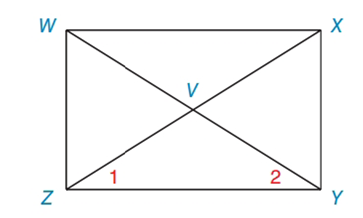Chapter 4.3, Problem 26EElementary Geometry For College St...

7th Edition
Alexander + 2 others
ISBN: 9781337614085

Solutions

Chapter
SectionElementary Geometry For College St...

7th Edition
Alexander + 2 others
ISBN: 9781337614085
Textbook Problem

In Exercises 25 and 26, supply the missing statements and reasons. Given: ▭ W X Y Z  with diagonals  W Y ¯  and  X Z ¯ Prove: ∠ 1 ≅ ∠ 2PROOF Statements Reasons 1. ? 1. Given 2. ? 2. The diagonals of a rectangle are ≅ 3. W Z ¯ ≅ X Y ¯ 3. The opposite sides of a rectangle are ≅ 4. Z Y ¯ ≅ Z Y ¯ 4. ? 5. △ X Z Y ≅ △ W Y Z 5. ? 6. ? 6. ?

To determine

To supply:

The missing statements and reasons and prove 12.

Explanation

SSS Rule for triangle congruency:

Calculation:

Consider the rectangle.

 PROOF Statements Reasons 1. WXYZ is a rectangle with diagonals of WY and XZ. 1. Given 2. WY is congruent to XZ.WY¯≅ZX¯ 2. The diagonals of a rectangle are ≅ 3

Still sussing out bartleby?

Check out a sample textbook solution.

See a sample solution

The Solution to Your Study Problems

Bartleby provides explanations to thousands of textbook problems written by our experts, many with advanced degrees!

Get Started

let f(x) = x 1, g(x) = x+1, and h(x) = 2x3 1. Find the rule for each function. 13. gh

Applied Calculus for the Managerial, Life, and Social Sciences: A Brief Approach

True or False: is a convergent series.

Study Guide for Stewart's Single Variable Calculus: Early Transcendentals, 8th

The area of the shaded region is given by:

Study Guide for Stewart's Multivariable Calculus, 8th